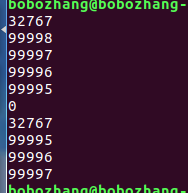c语言冒泡排序的结果中突然出现数据变成0

#include
#include
#define N 5

int number[N]={99999,99998,99997,99996,99995};
int num;

void pr(){
int i=0;
for(i=0;i printf("%d\n",number[i]);
}
}
void sort_order(){
int i,k;
for(i=0;i for(k=0;k if(number[k]>=number[k+1]){
num = number[k];
number[k] = number[k+1];
number[k+1] = num;
}
}
}
pr();
}

int main(int argc, char const *argv[])
{
number=32767;
pr();
sort_order();
return 0;
}1个回答

`````` for(k=0;k<N-i;k++){
->
for(k=0;k<N-i-1;k++){
``````